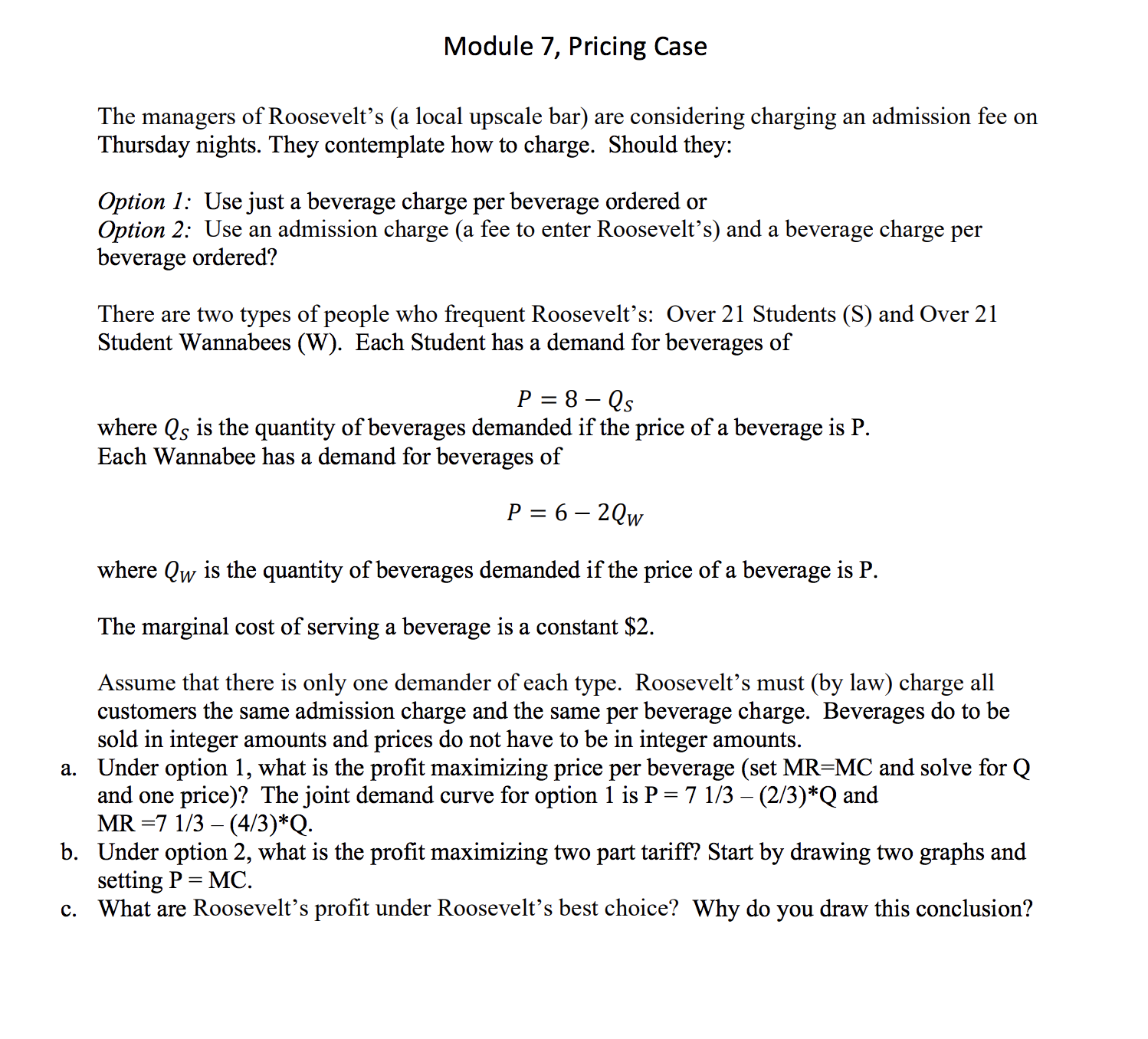# Module 7, Pricing CaseThe managers of Roosevelt's (a local upscale bar)Thursday nights. They contemplate how to charge. Should they:are considering chargingan admission fee onSOption 1: Use just a beverage charge per beverage ordered orOption 2: Use an admission charge (a fee to enter Roosevelt's) and a beverage charge perbeverage ordered?There are two types of people who frequent Roosevelt's: Over 21 Students (S) and Over 21Student Wannabees (W). Each Student has a demand for beverages of8 - QsPwhere Qs is the quantity of beverages demanded if the price of a beverage is PEach Wannabee has a demand for beverages ofP 6-2Qwwhere Qw is the quantity of beverages demanded if the price of a beverage is PThe marginal cost of serving a beverage is a constant \$2Assume that there is onlycustomers the same admission charge and the same per beverage charge. Beverages do to besold in integer amounts and prices do not have to be in integer amounts.a. Under option 1, what is the profit maximizing price per beverage (set MR-MC and solve for Qand one price)? The joint demand curve for option 1 is P = 7 1/3 - (2/3)*Q andMR 7 1/3 (4/3)*Qb. Under option 2, what is the profit maximizing two part tariff? Start by drawing two graphs andsetting P MC.c. What are Roosevelt's profit under Roosevelt's best choice? Why do you draw this conclusion?one demander of each type. Roosevelt's must (by law) charge all

Questionhelp_outlineImage TranscriptioncloseModule 7, Pricing Case The managers of Roosevelt's (a local upscale bar) Thursday nights. They contemplate how to charge. Should they: are considering charging an admission fee on S Option 1: Use just a beverage charge per beverage ordered or Option 2: Use an admission charge (a fee to enter Roosevelt's) and a beverage charge per beverage ordered? There are two types of people who frequent Roosevelt's: Over 21 Students (S) and Over 21 Student Wannabees (W). Each Student has a demand for beverages of 8 - Qs P where Qs is the quantity of beverages demanded if the price of a beverage is P Each Wannabee has a demand for beverages of P 6-2Qw where Qw is the quantity of beverages demanded if the price of a beverage is P The marginal cost of serving a beverage is a constant \$2 Assume that there is only customers the same admission charge and the same per beverage charge. Beverages do to be sold in integer amounts and prices do not have to be in integer amounts. a. Under option 1, what is the profit maximizing price per beverage (set MR-MC and solve for Q and one price)? The joint demand curve for option 1 is P = 7 1/3 - (2/3)*Q and MR 7 1/3 (4/3)*Q b. Under option 2, what is the profit maximizing two part tariff? Start by drawing two graphs and setting P MC. c. What are Roosevelt's profit under Roosevelt's best choice? Why do you draw this conclusion? one demander of each type. Roosevelt's must (by law) charge all fullscreen
check_circleExpert Solution
Step 1

Using just the beverage charge per unit:

MR= 71/3- (4/3)*Q , P =  71/3- (2/3)*Q

For MR=MC=\$2:

71/3 – (4/3)Q=2

Q= 25.667 *(3/4)=

Therefore, joint quantity and price are:

Q=19.25units

P= \$10.83

Step 2

b) Two-part tariff is a type of price discrimination, which a monopolist uses wherein the price of the good or service is composed of two types a lumpsum fee and a per-unit charge of beverage.

Step 3

Student demand: (MC=P)

P=8-Qs=2

Q2= 6 units , Maximum willingness to pay = \$8

Consumer ...

### Want to see the full answer?

See Solution

#### Want to see this answer and more?

Solutions are written by subject experts who are available 24/7. Questions are typically answered within 1 hour*

See Solution
*Response times may vary by subject and question
Tagged in